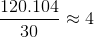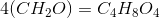# College Chemistry : Combustion Reactions

## Example Questions

### Example Question #1 : Redox Reactions

Suppose that the only elements a compound contains are carbon, hydrogen, and oxygen. If complete combustion of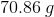of this compound produces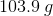of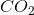and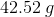of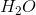, what is this compound's molecular formula?

Note: The molecular weight of this compound is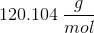.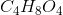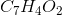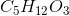Explanation:

To answer this question, it's important to remember the details of combustion reactions. In the presence of oxygen and a sufficient amount of energy to initiate the process, combustion reactions that go to completion will result in the complete oxidation and breakdown of the initial reactant.

Theproduced from the reaction contains all of the carbon in the original reactant. Likewise, theproduced contains all the hydrogen in the original compound. The oxygen content in the original compound is everything left over.

The first step then is to use the mass of each product to find the mass of each element in the original compound. To do this, we'll need to use the molar mass for each of the products as well as the individual elements.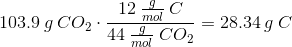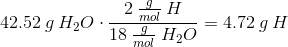The combined mass of carbon and hydrogen in the original compound is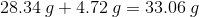.

The remaining mass will come from oxygen. Thus, the original compound will contain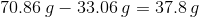of oxygen.

Once we have the mass of each element, we can use that information to calculate the number of moles of each element in the original compound.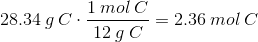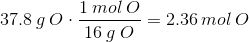Now that we have the relative molar amounts of each element in the original compound, we can find the empirical formula by finding the smallest whole number ratios.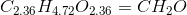From this, we can conclude that the empirical mass of the compound is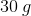.

Finally, since we are given the compound's molecular weight, we can use that information to find the molecular formula for the compound.